# 朴实无华，图解快排，多语言实现。（PS：还有宝藏资料）

## 二、算法思想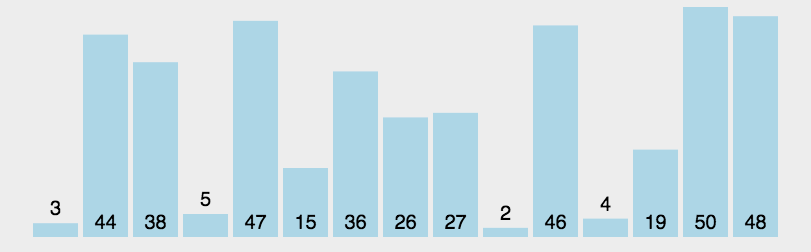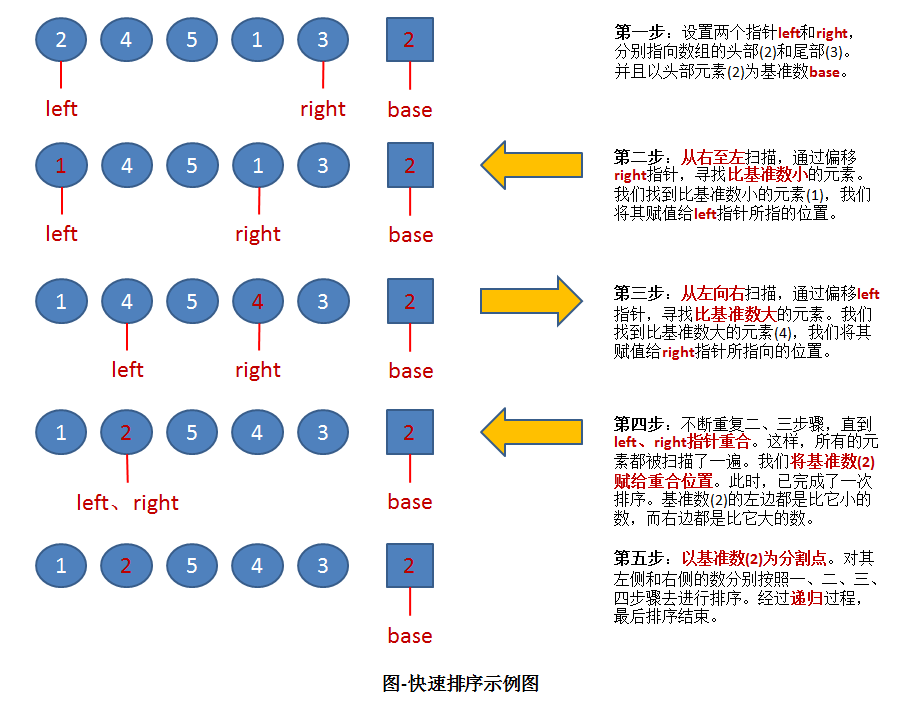2左边的数组只有一个元素1，所以显然不用再排序，位置也被确定。（注：这种情况时，left指针和right指针显然是重合的。因此在代码中，我们可以通过设置判定条件left必须小于right，如果不满足，则不用排序了）。

### 1、代码

C++：

#include <iostream>
#include <vector>

using namespace std;

int division(vector<int> &list, int left, int right){
// 以最左边的数(left)为基准
int base = list[left];
while (left < right) {
// 从序列右端开始，向左遍历，直到找到小于base的数
while (left < right && list[right] >= base)
right--;
// 找到了比base小的元素，将这个元素放到最左边的位置
list[left] = list[right];

// 从序列左端开始，向右遍历，直到找到大于base的数
while (left < right && list[left] <= base)
left++;
// 找到了比base大的元素，将这个元素放到最右边的位置
list[right] = list[left];
}

// 最后将base放到left位置。此时，left位置的左侧数值应该都比left小；
// 而left位置的右侧数值应该都比left大。
list[left] = base;
return left;
}

// 快速排序
void QuickSort(vector<int> &list, int left, int right){
// 左下标一定小于右下标，否则就越界了
if (left < right) {
// 对数组进行分割，取出下次分割的基准标号
int base = division(list, left, right);

// 对“基准标号“左侧的一组数值进行递归的切割，以至于将这些数值完整的排序
QuickSort(list, left, base - 1);

// 对“基准标号“右侧的一组数值进行递归的切割，以至于将这些数值完整的排序
QuickSort(list, base + 1, right);
}
}

void main(){
int arr[] = { 6, 4, 8, 9, 2, 3, 1 };
vector<int> test(arr, arr + sizeof(arr) / sizeof(arr));
cout << "排序前" << endl;
for (int i = 0; i < test.size(); i++){
cout << test[i] << " ";
}
cout << endl;
vector<int> result = test;
QuickSort(result, 0, result.size() - 1);
cout << "排序后" << endl;
for (int i = 0; i < result.size(); i++){
cout << result[i] << " ";
}
cout << endl;
system("pause");
}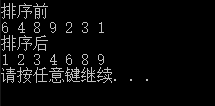Python：

# -*- coding:utf-8 -*-

def QuickSort(input_list, left, right):
'''
函数说明:快速排序（升序）
Author:
www.cuijiahua.com
Parameters:
input_list - 待排序列表
Returns:
无
'''
def division(input_list, left, right):
'''
函数说明:根据left和right进行一次扫描，重新找到基准数
Author:
www.cuijiahua.com
Parameters:
input_list - 待排序列表
left - 左指针位置
right - 右指针位置
Returns:
left - 新的基准数位置
'''
base = input_list[left]
while left < right:
while left < right and input_list[right] >= base:
right -= 1
input_list[left] = input_list[right]
while left < right and input_list[left] <= base:
left += 1
input_list[right] = input_list[left]
input_list[left] = base
return left

if left < right:
base_index = division(input_list, left, right)
QuickSort(input_list, left, base_index - 1)
QuickSort(input_list, base_index + 1, right)

if __name__ == '__main__':
input_list = [6, 4, 8, 9, 2, 3, 1]
print('排序前:', input_list)
QuickSort(input_list, 0, len(input_list) - 1)
print('排序后:', input_list)

## 三、算法分析

### 1、快速排序算法的性能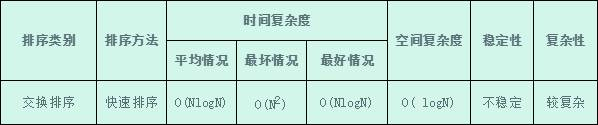### 4、算法稳定性

Jack-Cui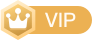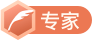CSDN认证博客专家 算法工程师04-1766
08-241963
01-28384
02-05335
12-253262
04-15106
09-057万+
04-02
05-313881
09-148312
04-29764
09-245万+
04-291万+
06-041276
12-16106
09-0543
©️2020 CSDN 皮肤主题: 技术黑板 设计师:CSDN官方博客